Theory and Modern Applications

# On the transcendence of some power series

## Abstract

In this study, we consider some power series with rational coefficients and investigate transcendence of the values of these series for Liouville number arguments. It is proved that these values are either a Liouville number or a rational number under certain conditions.

AMS Subject Classification:11J81, 11J17.

## 1 Introduction

A real number α is algebraic if it is a zero of a polynomial with integer coefficients. The real numbers which are not algebraic are known as transcendental. The theory of transcendental numbers has a long history and was originated back to Liouville in his famous paper  where he explicitly constructed a number and proved that it is transcendental. Later, Cantor  gave another proof of the existence of transcendental numbers by establishing the denumerability of the set of algebraic numbers. It follows from this that almost all real numbers are transcendental. Further, the development of the theory of transcendental numbers has proved to have a strong influence on some new studies in Diophantine equations; see [3, 4].

A classification of the set of all transcendental numbers into three disjoint classes, termed S, T and U, which was introduced by Mahler , proved to be of considerable value in the general development of the subject. The first classification of this kind was outlined by Maillet in , and others were described by Perna in  and Morduchai-Boltovskoj , but Mahler’s classification receives most of the interest. Mahler described this classification in the following way.

Let $P\left(x\right)={a}_{n}{x}^{n}+\cdots +{a}_{1}x+{a}_{0}$ be a polynomial with integer coefficients. The height $H\left(P\right)$ of the polynomial P is defined by

$H\left(P\right)=\underset{n}{max}\left(|{a}_{n}|,\dots ,|{a}_{0}|\right)$

and if the degree of P is denoted by $deg\left(P\right)$, then $deg\left(P\right)=n$, and for a given arbitrary complex number ξ, it can be written as

${\omega }_{n}\left(H,\xi \right)=\underset{n}{min}\left\{|P\left(\xi \right)|:deg\left(P\right)\le n,H\left(P\right)\le H,P\left(\xi \right)\ne 0\right\},$

where n and H are positive integers; see . Next, Mahler puts

${\omega }_{n}\left(\xi \right)=\underset{H\to \mathrm{\infty }}{\stackrel{‾}{lim}}\frac{-log{\omega }_{n}\left(H,\xi \right)}{logH}$

and

$\omega \left(\xi \right)=\underset{n\to \mathrm{\infty }}{\stackrel{‾}{lim}}\frac{{\omega }_{n}\left(\xi \right)}{n}.$

The inequalities $0\le {\omega }_{n}\left(\xi \right)\le \mathrm{\infty }$ and $0\le \omega \left(\xi \right)\le \mathrm{\infty }$ hold. From ${\omega }_{n+1}\left(H,\xi \right)\le {\omega }_{n}\left(H,\xi \right)$, we get ${\omega }_{n+1}\left(\xi \right)\ge {\omega }_{n}\left(\xi \right)$. If index ${\omega }_{n}\left(\xi \right)=\mathrm{\infty }$, then the $\mu \left(\xi \right)$ is defined as the smallest of them; otherwise $\mu \left(\xi \right)=\mathrm{\infty }$. Thus, $\mu \left(\xi \right)$ is uniquely determined. Furthermore, the two quantities $\mu \left(\xi \right)$ and $\omega \left(\xi \right)$ are never finite simultaneously, for the finiteness of $\mu \left(\xi \right)$ implies that there is an $n<\mathrm{\infty }$ such that ${\omega }_{n}=\mathrm{\infty }$, hence $\omega =\mathrm{\infty }$.

Therefore, there are the following four possibilities for ξ: it is said to be

In , Koksma introduced an analogous classification of complex numbers. He divided the complex numbers into four classes ${A}^{\ast }$, ${S}^{\ast }$, ${T}^{\ast }$ and ${U}^{\ast }$. More information may be found in .

In , Wirsing proved that both classifications are equivalent. Namely, A, S, T and U numbers are the same as ${A}^{\ast }$, ${S}^{\ast }$, ${T}^{\ast }$ and ${U}^{\ast }$ numbers. The class A is precisely the set of algebraic numbers. The ξ is called a U-number of degree m if $\mu \left(\xi \right)=m$. The set of U-numbers of degree m is denoted by ${U}_{m}$. It is obvious that for any $m\ge 1$, the ${U}_{m}$ is a subclass of U, and U is the union of all disjoint sets ${U}_{m}$.

Leveque proved that ${U}_{m}$ is not empty for any $m\ge 1$ in . Later, in [13, 14], Oryan considered a class of power series with algebraic coefficients and proved that under certain conditions these series take values in the subclass ${U}_{m}$ for algebraic arguments and in the set of Liouville numbers for Liouville number arguments, respectively.

Now, consider the infinite convergent sum $T={\sum }_{n=0}^{\mathrm{\infty }}\frac{P\left(n\right)}{Q\left(n\right)}$, where $P\left(x\right)\in \overline{\mathbb{Q}}\left[x\right]$, $Q\left(x\right)\in \mathbb{Q}\left[x\right]$ and $Q\left(x\right)$ has only simple rational zeros. Then, Saradha and Tijdeman have obtained the sufficient and necessary conditions for the transcendence of T if the degree of $Q\left(x\right)$ is 3; see . Similarly, Ping and Yuan gave sufficient and necessary conditions for the transcendence of T if the degree of $Q\left(x\right)$ is 4 and $Q\left(x\right)$ is reduced; see .

We also note that a transcendental function is an analytic function having a single value or many values, and to calculate the values, we need a limiting process. However, an analytic function is transcendental if and only if its Riemann surface is non-compact; see .

In the present work, we consider certain power series with rational coefficients and show that these series take values of either the set of Liouville numbers or rational numbers under certain conditions. Thus, we give a new result for obtaining ${U}_{1}$-numbers.

## 2 Preliminaries

In this paper, $|x|$ means the absolute value of x and the least common multiple of ${x}_{1},{x}_{2},\dots ,{x}_{n}$ is denoted by $\left[{x}_{1},{x}_{2},\dots ,{x}_{n}\right]$.

Definition 2.1 A real number ξ is called Liouville number if and only if for every positive integer n, there exist integers ${p}_{n}$, ${q}_{n}$ (${q}_{n}>1$) with

$0<|\xi -\frac{{p}_{n}}{{q}_{n}}|<\frac{1}{{{q}_{n}}^{n}}.$

The set of all Liouville numbers is identical with the ${U}_{1}$ subclass. More information about Liouville numbers may be found in [18, 19] and .

Now, in order to prove our main theorem, we need the following lemma which was proved in .

Lemma 2.2 Let ξ be a real number. If there exist a sequence ${\omega }_{1},{\omega }_{2},{\omega }_{3},\dots$ of real numbers tending to infinity and a sequence $\frac{{p}_{1}}{{q}_{1}},\frac{{p}_{2}}{{q}_{2}},\frac{{p}_{3}}{{q}_{3}},\dots$ of rational numbers satisfying

$0<|\xi -\frac{{p}_{n}}{{q}_{n}}|<\frac{1}{{{q}_{n}}^{{\omega }_{n}}}\phantom{\rule{1em}{0ex}}\left({q}_{n}>1,n=1,2,3,\dots \right),$

then ξ is a Liouville number.

## 3 Main theorem

Theorem 3.1 Let

$g\left(x\right)=\sum _{n=0}^{\mathrm{\infty }}\frac{{f}_{n}}{{e}_{n}}{x}^{n}$

be a power series with non-zero rational coefficients $\frac{{f}_{n}}{{e}_{n}}$ (${e}_{n},{f}_{n}\in \mathbb{Z}$, ${e}_{n}>1$) which satisfies the following conditions: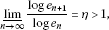(3.1)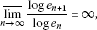(3.2)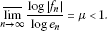(3.3)

Further, let ξ be a Liouville number and satisfy the following two properties:

1. (1)

The ξ has an approximation with rational numbers $\frac{{p}_{n}}{{q}_{n}}$ (${q}_{n}>1$) so that the following inequality holds for sufficiently large n:

$|\xi -\frac{{p}_{n}}{{q}_{n}}|<\frac{1}{{{q}_{n}}^{n{s}_{n}}}\phantom{\rule{1em}{0ex}}\left(\underset{n\to \mathrm{\infty }}{lim}{s}_{n}=+\mathrm{\infty }\right).$
(3.4)
2. (2)

There exist two positive real numbers ${\gamma }_{1}$ and ${\gamma }_{2}$ with $\frac{\eta }{\eta -1}<{\gamma }_{1}<{\gamma }_{2}$ and

${e}_{n}^{{\gamma }_{1}}\le {q}_{n}^{n}\le {e}_{n}^{{\gamma }_{2}}$
(3.5)

for sufficiently large n.

Then $g\left(\xi \right)$ is either a Liouville number or a rational number.

Proof It follows from (3.1) that

$log{e}_{n+1}>{\eta }_{1}log{e}_{n}$
(3.6)

for sufficiently large n, where ${\eta }_{1}=\eta -{\epsilon }_{1}$ and ${\epsilon }_{1}$ is chosen as $0<{\epsilon }_{1}<\eta -\frac{{\gamma }_{1}}{{\gamma }_{1}-1}$. Then it follows from (3.6) that the sequence $\left\{{e}_{n}\right\}$ is strictly increasing, thus we have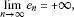(3.7)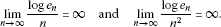(3.8)

Now, by using equation (3.6), we get

${e}_{n}<{e}_{n+1}^{\frac{1}{{\eta }_{1}}}.$
(3.9)

Let ${E}_{n}=\left[{e}_{0},{e}_{1},\dots ,{e}_{n}\right]$. Then on using (3.9), we obtain

${e}_{n}\le {E}_{n}\le {e}_{n}^{{\epsilon }_{2}+\frac{{\eta }_{1}}{{\eta }_{1}-1}},$
(3.10)

where ${\epsilon }_{2}$ is chosen as $0<{\epsilon }_{2}<{\gamma }_{1}-\frac{{\eta }_{1}}{{\eta }_{1}-1}$.

Now, if we consider the following polynomials:

${g}_{n}\left(x\right)=\sum _{\nu =0}^{n}\frac{{f}_{\nu }}{{e}_{\nu }}{x}^{\nu }\phantom{\rule{1em}{0ex}}\left(n=1,2,3,\dots \right),$

then we have

${g}_{n}\left(\xi \right)-{g}_{n}\left(\frac{{p}_{n}}{{q}_{n}}\right)=\sum _{\nu =1}^{n}\frac{{f}_{\nu }}{{e}_{\nu }}\left(\xi -\frac{{p}_{n}}{{q}_{n}}\right)\left({\xi }^{\nu -1}+{\xi }^{\nu -2}\frac{{p}_{n}}{{q}_{n}}+\cdots +\frac{{p}_{n}^{\nu -1}}{{q}_{n}^{\nu -1}}\right),$
(3.11)

and from (3.4) it follows that

$|\frac{{p}_{n}}{{q}_{n}}|\le |\xi |+1.$
(3.12)

Further, on using equations (3.4), (3.11) and (3.12), we get

$|{g}_{n}\left(\xi \right)-{g}_{n}\left(\frac{{p}_{n}}{{q}_{n}}\right)|\le \frac{1}{{q}_{n}^{n{s}_{n}}}\sum _{\nu =1}^{n}\frac{|{f}_{\nu }|}{{e}_{\nu }}\nu {\left(|\xi |+1\right)}^{\nu -1}.$
(3.13)

Now, define

${F}_{n}=\underset{n}{max}\left(|{f}_{0}|,|{f}_{1}|,\dots ,|{f}_{n}|\right).$

Then we obtain

$\sum _{\nu =1}^{n}\frac{|{f}_{\nu }|}{{e}_{\nu }}\nu {\left(|\xi |+1\right)}^{\nu -1}\le {n}^{2}{F}_{n}{\left(|\xi |+1\right)}^{n-1}$
(3.14)

for sufficiently large n.

On the other hand, we can easily deduce from (3.3) and $\mu <\frac{\mu +1}{2}$ that

$\frac{log|{f}_{n}|}{log{e}_{n}}<\frac{\mu +1}{2},$
(3.15)

and since the sequence $\left\{{e}_{n}\right\}$ is strictly increasing, then it follows that

$\frac{log{F}_{n}}{log{e}_{n}}<\frac{\mu +1}{2}.$
(3.16)

On using $0<{\epsilon }_{2}<{\gamma }_{1}-\frac{{\eta }_{1}}{{\eta }_{1}-1}$, and from (3.7), (3.8) and (3.16), we get

${n}^{2}{F}_{n}{\left(|\xi |+1\right)}^{n-1}\le \frac{1}{2}{e}_{n}^{\left({\gamma }_{1}-{\epsilon }_{2}-\frac{{\eta }_{1}}{{\eta }_{1}-1}\right)\frac{{s}_{n}}{2}}.$

That is, on using (3.5), (3.10) and (3.14),

$\sum _{\gamma =1}^{n}\frac{|{f}_{\gamma }|}{{e}_{\gamma }}\gamma {\left(|\xi |+1\right)}^{\gamma -1}\le \frac{{\left({q}_{n}^{n}\right)}^{\frac{{s}_{n}}{2}}}{2{\left({E}_{n}\right)}^{\frac{{s}_{n}}{2}}}$

for sufficiently large n. Then on using (3.13), we have

$|{g}_{n}\left(\xi \right)-{g}_{n}\left(\frac{{p}_{n}}{{q}_{n}}\right)|\le \frac{1}{2{\left({E}_{n}{q}_{n}^{n}\right)}^{\frac{{s}_{n}}{2}}}$
(3.17)

for sufficiently large n.

Moreover, the following inequality holds:

$|g\left(\xi \right)-{g}_{n}\left(\xi \right)|\le \sum _{i=1}^{\mathrm{\infty }}\frac{|{f}_{n+i}|}{{e}_{n+i}}|\xi {|}^{n+i}.$
(3.18)

It follows from (3.15) that

$\frac{|{f}_{n}|}{{e}_{n}}<\frac{1}{{e}_{n}^{\left(\frac{1-\mu }{2}\right)}}$

for sufficiently large n. We get from here and (3.18)

$|g\left(\xi \right)-{g}_{n}\left(\xi \right)|\le \frac{|\xi {|}^{n+1}}{{e}_{n+1}^{\left(\frac{1-\mu }{2}\right)}}\left[1+{\left(\frac{{e}_{n+1}}{{e}_{n+2}}\right)}^{\frac{1-\mu }{2}}|\xi |+{\left(\frac{{e}_{n+1}}{{e}_{n+3}}\right)}^{\frac{1-\mu }{2}}|\xi {|}^{2}+\cdots \right].$

Thus, we can deduce from (3.9) that

$0<\frac{{e}_{n+1}}{{e}_{n+2}}<\frac{1}{{e}_{n+2}^{\left(1-\frac{1}{{\eta }_{1}}\right)}}.$

Since (3.7) holds, we then obtain

$\underset{n\to \mathrm{\infty }}{lim}\frac{{e}_{n+1}}{{e}_{n+2}}=0.$

Similarly, since $\frac{1-\mu }{2}>0$, we have

${\left(\frac{{e}_{n+1}}{{e}_{n+1+k}}\right)}^{\frac{1-\mu }{2}}|\xi {|}^{k}<{\left(\frac{1}{2}\right)}^{k}\phantom{\rule{1em}{0ex}}\left(k=1,2,3,\dots \right),$

and therefore

$|g\left(\xi \right)-{g}_{n}\left(\xi \right)|\le \frac{2|\xi {|}^{n+1}}{{e}_{n+1}^{\left(\frac{1-\mu }{2}\right)}}.$

On the other hand, from (3.8) we get

$2|\xi {|}^{n+1}\le {e}_{n+1}^{\left(\frac{1-\mu }{4}\right)}$

for sufficiently large n. From here we obtain

$|g\left(\xi \right)-{g}_{n}\left(\xi \right)|\le \frac{1}{{e}_{n+1}^{\left(\frac{1-\mu }{4}\right)}}$
(3.19)

for sufficiently large n. Now, if further we define

${r}_{n}=\frac{log{e}_{n+1}}{log{e}_{n}},$

then we have

$|g\left(\xi \right)-{g}_{n}\left(\xi \right)|\le \frac{1}{{e}_{n}^{{r}_{n}\left(\frac{1-\mu }{4}\right)}}.$

Using (3.2) then it follows that there exists a subsequence $\left\{{r}_{{n}_{k}}\right\}$ of $\left\{{r}_{n}\right\}$ such that

$\underset{k\to \mathrm{\infty }}{lim}{r}_{{n}_{k}}=\mathrm{\infty }.$

Therefore,

$|g\left(\xi \right)-{g}_{{n}_{k}}\left(\xi \right)|\le \frac{1}{{e}_{{n}_{k}}^{{r}_{{n}_{k}}\left(\frac{1-\mu }{4}\right)}}$
(3.20)

for sufficiently large ${n}_{k}$. On using (3.5), (3.7) and (3.10), we deduce that there exists a suitable sequence $\left\{{r}_{{n}_{k}}^{\prime }\right\}$ with ${lim}_{k\to \mathrm{\infty }}{r}_{{n}_{k}}^{\prime }=+\mathrm{\infty }$. Then from (3.20) for ${r}_{{n}_{k}}^{\prime }$ we obtain

$\frac{1}{{e}_{{n}_{k}}^{{r}_{{n}_{k}}\left(\frac{1-\mu }{4}\right)}}\le \frac{1}{2{\left({E}_{{n}_{k}}{q}_{{n}_{k}}^{{n}_{k}}\right)}^{{{r}^{\mathrm{\prime }}}_{{n}_{k}}}},$

and therefore

$|g\left(\xi \right)-{g}_{{n}_{k}}\left(\xi \right)|\le \frac{1}{2{\left({E}_{{n}_{k}}{q}_{{n}_{k}}^{{n}_{k}}\right)}^{{{r}^{\mathrm{\prime }}}_{{n}_{k}}}}$
(3.21)

for sufficiently large ${n}_{k}$. On the other hand, using (3.17) we get

$|{g}_{{n}_{k}}\left(\xi \right)-{g}_{{n}_{k}}\left(\frac{{p}_{{n}_{k}}}{{q}_{{n}_{k}}}\right)|\le \frac{1}{2{\left({E}_{{n}_{k}}{q}_{{n}_{k}}^{{n}_{k}}\right)}^{\frac{{s}_{{n}_{k}}}{2}}}$
(3.22)

for sufficiently large ${n}_{k}$.

Let

${\omega }_{{n}_{k}}=\underset{k}{min}\left({r}_{{n}_{k}}^{\prime },\frac{{s}_{{n}_{k}}}{2}\right).$

It follows from (3.21) and (3.22) that

$|g\left(\xi \right)-{g}_{{n}_{k}}\left(\frac{{p}_{{n}_{k}}}{{q}_{{n}_{k}}}\right)|\le \frac{1}{{\left({E}_{{n}_{k}}{q}_{{n}_{k}}^{{n}_{k}}\right)}^{{\omega }_{{n}_{k}}}},$
(3.23)

where ${lim}_{k\to \mathrm{\infty }}{\omega }_{{n}_{k}}=\mathrm{\infty }$. Moreover,

${g}_{{n}_{k}}\left(\frac{{p}_{{n}_{k}}}{{q}_{{n}_{k}}}\right)=\frac{{z}_{{n}_{k}}}{{E}_{{n}_{k}}{q}_{{n}_{k}}^{{n}_{k}}}$

are rational numbers with ${z}_{{n}_{k}}$ integers. It follows from (3.23) that

$\underset{k\to \mathrm{\infty }}{lim}{g}_{{n}_{k}}\left(\frac{{p}_{{n}_{k}}}{{q}_{{n}_{k}}}\right)=g\left(\xi \right).$

Thus, if the sequence $\left\{{g}_{{n}_{k}}\left(\frac{{p}_{{n}_{k}}}{{q}_{{n}_{k}}}\right)\right\}$ is constant, then $g\left(\xi \right)$ is a rational number. Otherwise, using Lemma 2.2 we get from (3.23) that $g\left(\xi \right)$ is a Liouville number. □

Corollary 3.2 If ${f}_{n}>0$ ($n=0,1,2,\dots$) and $\xi >0$ in Theorem  3.1, then $f\left(\xi \right)$ is a Liouville number.

Proof Since $\xi >0$, it is possible to choose a subsequence $\left\{\frac{{p}_{{{n}_{k}}_{t}}}{{q}_{{{n}_{k}}_{t}}}\right\}$ of $\left\{\frac{{p}_{{n}_{k}}}{{q}_{{n}_{k}}}\right\}$ so that the terms $\frac{{p}_{{{n}_{k}}_{t}}}{{q}_{{{n}_{k}}_{t}}}$ are positive and strictly increasing or decreasing. Let us assume that $\left\{\frac{{p}_{{{n}_{k}}_{t}}}{{q}_{{{n}_{k}}_{t}}}\right\}$ is strictly increasing. From $\frac{{f}_{n}}{{e}_{n}}>0$ it follows

${g}_{{{n}_{k}}_{t+1}}\left(\frac{{p}_{{{n}_{k}}_{t+1}}}{{q}_{{{n}_{k}}_{t+1}}}\right)-{g}_{{{n}_{k}}_{t}}\left(\frac{{p}_{{{n}_{k}}_{t}}}{{q}_{{{n}_{k}}_{t}}}\right)>0.$
(3.24)

From (3.24) we deduce that the sequence $\left\{{g}_{{n}_{k}}\left(\frac{{p}_{{n}_{k}}}{{q}_{{n}_{k}}}\right)\right\}$ is not constant. Thus, $g\left(\xi \right)$ is a Liouville number. In the case of strictly decreasing $\left\{\frac{{p}_{{{n}_{k}}_{t}}}{{q}_{{{n}_{k}}_{t}}}\right\}$, the proof follows similarly. □

## 4 Conclusion

In this paper, the series with rational coefficients are treated and it is shown that under certain conditions these series take values belonging to either the set of Liouville numbers or the rational number field for Liouville number arguments.

The similar results can be proved for the power series which are defined in the p-adic field ${Q}_{p}$ and in the field of formal Laurent series.

## References

1. Liouville J: Sur des classes trés étendues de quantités dont la valeur n’est ni algébrique, ni méme reductible à des irrationnelles algébriques. C. R. Math. Acad. Sci. Paris 1844, 18: 883-885. 910-911

2. Cantor G: Über eine Eigenschaft des Inbegriffs aller reelen algebraishen Zahlen. J. Reine Angew. Math. 1974, 77: 258-262.

3. Baker A: Transcendental Number Theory. Cambridge University Press, Cambridge; 1975.

4. Sprindzhuk VG: Classical Diophantine Equations in Two Unknowns. Nauka, Moscow; 1982. (In Russian)

5. Mahler K: Zur Approximation der Exponentialfunktion und des Logarithmus, I, II. J. Reine Angew. Math. 1932, 166: 118-150.

6. Maillet E: Sur la classification des irrationelles. C. R. Math. Acad. Sci. Paris 1906, 143: 26-28.

7. Perna A: Sui numeri transcendenti in generale e sulla loroconstruzione in base al criterio di Liouville. G. Mat. Battaglini 1914, 52: 305-365.

8. Morduchai-Boltovskoj D: On transcendental numbers with successive approximations defined by algebraic equations. Rec. Math. Moscou 1934, 41: 221-232.

9. Koksma JF: Über die Mahlersche Klasseneinteilung der transzendenten Zahlen und die Approximation komplexer Zahlen durch algebraische Zahlen. Monatshefte Math. Phys. 1939, 48: 176-189. 10.1007/BF01696176

10. Bugeaud Y Cambridge Tracts in Mathematics 160. In Approximation by Algebraic Numbers. Cambridge University Press, Cambridge; 2004.

11. Wirsing E: Approximation mit algebraischen Zahlen beschr änkten Grades. J. Reine Angew. Math. 1961, 206: 67-77.

12. Leveque WJ: On Mahler’s U -numbers. J. Lond. Math. Soc. 1953, 28: 220-229. 10.1112/jlms/s1-28.2.220

13. Oryan MH:Über gewisse Potenzreihen, die für algebraische Argumente Werte aus Der Mahlerschen Unterklassenas${U}_{m}$ nehmen. Istanbul Univ. Fen Fak. Mecm., Seri A 1980, 45: 1-42.

14. Oryan MH: Über gewisse Potenzreihen, deren Funktionswerte für Argumente aus der Menge der Liouvilleschen Zahlen U -Zahlen vom Grade ≤ m sind. Istanbul Univ. Fen Fak. Mecm., Seri A 1990, 47: 15-34.

15. Saradha N, Tijdeman R: On the transcendence of infinite sums of values of rational functions. J. Lond. Math. Soc. 2003, 67(3):580-592. 10.1112/S0024610702003988

16. Yuan P, Li J: On the transcendence of some infinite sums. J. Lond. Math. Soc. 2009, 80(2):431-445. 10.1112/jlms/jdp029

17. Mulase M, Penkava M: Ribbon graphs, quadratic differentials on Riemann surfaces, and algebraic curves defined over Q. Asian J. Math. 1998, 2: 875-920.

18. Leveque WJ II. In Topics in Number Theory. Addison-Wesley, Reading; 1956.

19. Perron O: Irrationalzahlen. Walter de Gruyter, Berlin; 1960.

20. Schneider T: Einführung in die transzendenten Zahlen. Springer, Berlin; 1957.

21. Mahler K: Lectures on Transcendental Numbers. Springer, Berlin; 1976.

## Acknowledgements

The authors express their sincere thanks to the referees for the careful and noteworthy reading of the manuscript and very helpful suggestions that improved the manuscript substantially. The first author acknowledges that this work was partially supported by Scientific Research Projects Coordination Unit of Istanbul University under project number 3414.

## Author information

Authors

### Corresponding author

Correspondence to Adem Kılıçman.

### Competing interests

The authors declare that they do not have any competing interest.

### Authors’ contributions

All the authors contributed equally.

## Rights and permissions

Open Access This article is distributed under the terms of the Creative Commons Attribution 2.0 International License ( https://creativecommons.org/licenses/by/2.0 ), which permits unrestricted use, distribution, and reproduction in any medium, provided the original work is properly cited.

Reprints and Permissions

Karadeniz Gözeri, G., Pekin, A. & Kılıçman, A. On the transcendence of some power series. Adv Differ Equ 2013, 17 (2013). https://doi.org/10.1186/1687-1847-2013-17